##### Quantum Physics For Dummies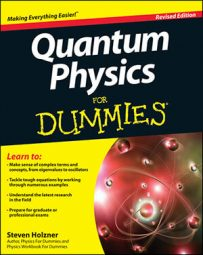In quantum physics, when you are working in one dimension, the general particle harmonic oscillator looks like the figure shown here, where the particle is under the influence of a restoring force — in this example, illustrated as a spring.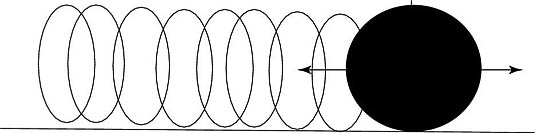A harmonic oscillator.

The restoring force has the form Fx = –kxx in one dimension, where kx is the constant of proportionality between the force on the particle and the location of the particle. The potential energy of the particle as a function of location x is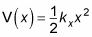This is also sometimes written as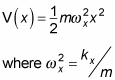Now take a look at the harmonic oscillator in three dimensions. In three dimensions, the potential looks like this: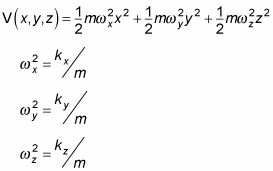Now that you have a form for the potential, you can start talking in terms of Schrödinger's equation: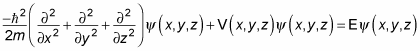Substituting in for the three-dimension potential, V(x, y, z), gives you this equation: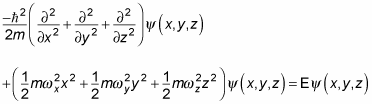Take this dimension by dimension. Because you can separate the potential into three dimensions, you can write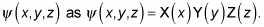Therefore, the Schrödinger equation looks like this for x: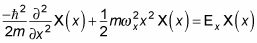Solving that equation, you get this next solution: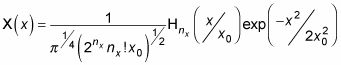where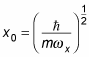and nx = 0, 1, 2, and so on. The Hnx term indicates a hermite polynomial, which looks like this:

• H0(x) = 1

• H1(x) = 2x

• H2(x) = 4x2 – 2

• H3(x) = 8x3 – 12x

• H4(x) = 16x4 – 48x2 + 12

• H5(x) = 32x5 – 160x3 + 120x

Therefore, you can write the wave function like this: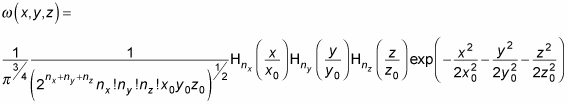That's a relatively easy form for a wave function, and it's all made possible by the fact that you can separate the potential into three dimensions.

What about the energy of the harmonic oscillator? The energy of a one-dimensional harmonic oscillator is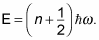And by analogy, the energy of a three-dimensional harmonic oscillator is given by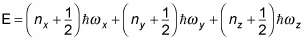Note that if you have an isotropic harmonic oscillator, where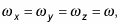the energy looks like this: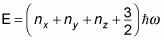As for the cubic potential, the energy of a 3D isotropic harmonic oscillator is degenerate. For example, E112 = E121 = E211. In fact, it's possible to have more than threefold degeneracy for a 3D isotropic harmonic oscillator — for example, E200 = E020 = E002 = E110 = E101 = E011.

In general, the degeneracy of a 3D isotropic harmonic oscillator is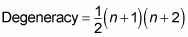where n = nx + ny + nz.